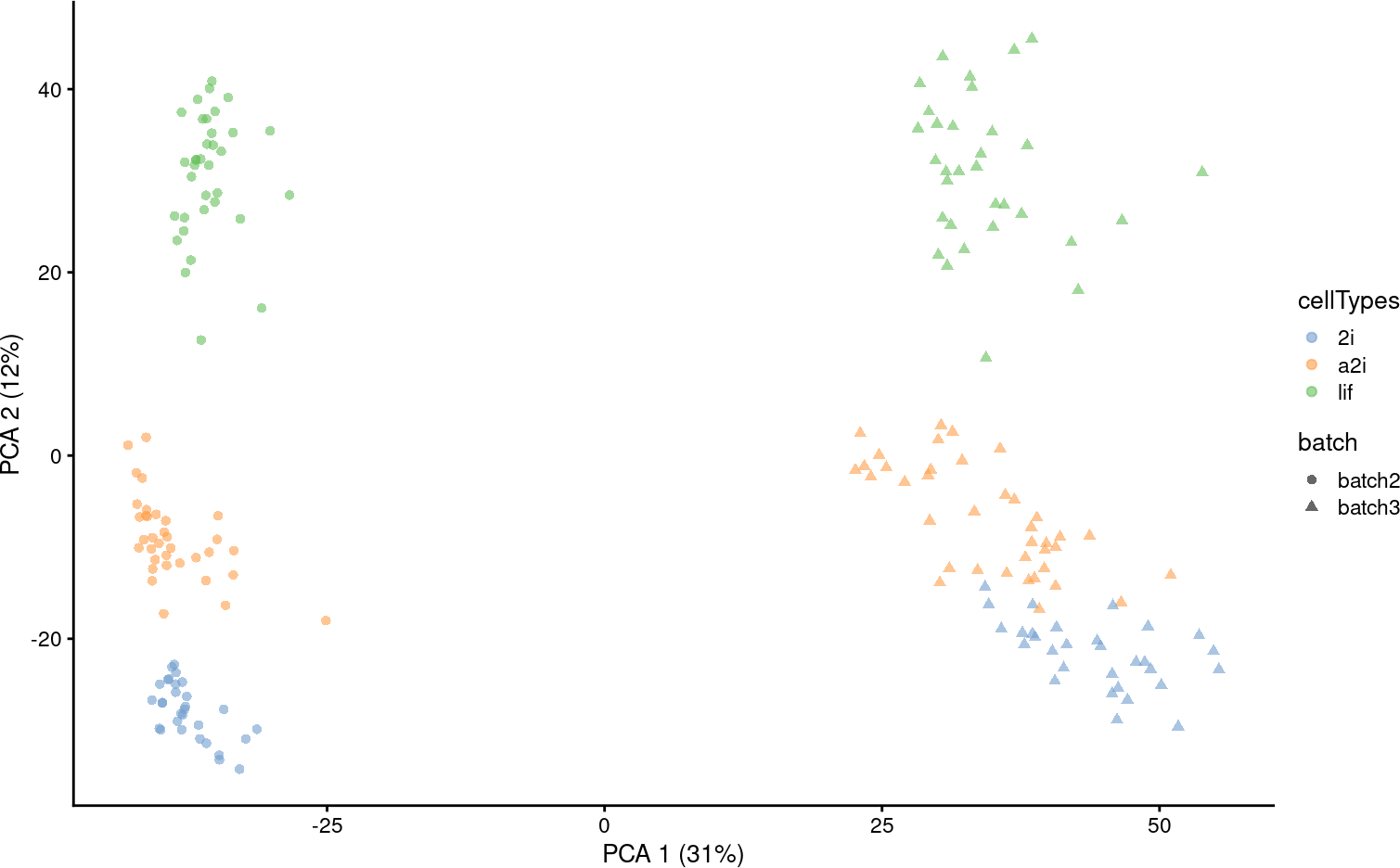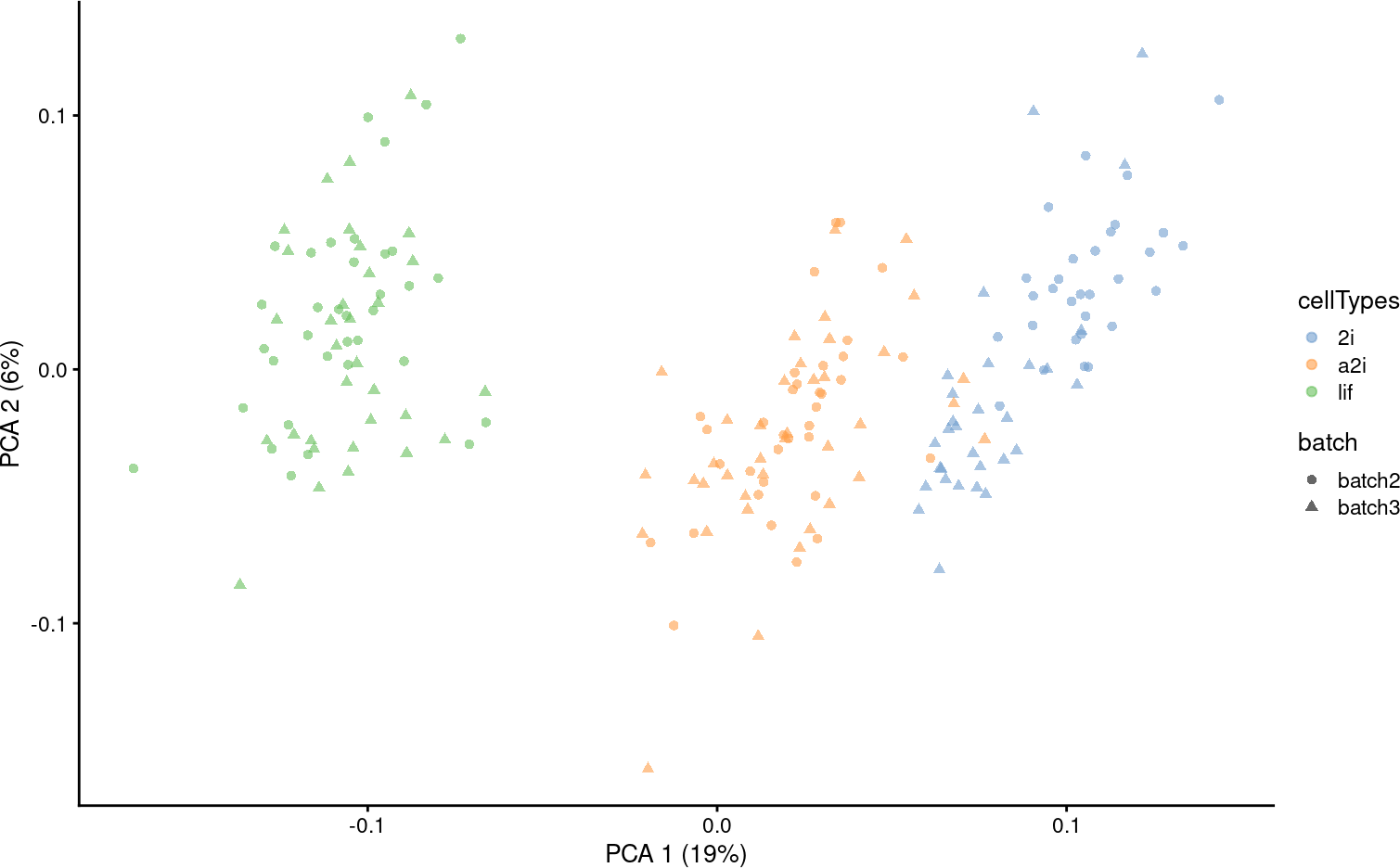# 1 Introduction

The scMerge algorithm allows batch effect removal and normalisation for single cell RNA-Seq data. It comprises of three key components including:

1. The identification of stably expressed genes (SEGs) as “negative controls” for estimating unwanted factors;
2. The construction of pseudo-replicates to estimate the effects of unwanted factors; and
3. The adjustment of the datasets with unwanted variation using a fastRUVIII model.

The purpose of this vignette is to illustrate some uses of `scMerge` and explain its key components.

We will load the `scMerge` package. We designed our package to be consistent with the popular BioConductor’s single cell analysis framework, namely the `SingleCellExperiment` and `scater` package.

``````suppressPackageStartupMessages({
library(SingleCellExperiment)
library(scMerge)
library(scater)
})``````

We provided an illustrative mouse embryonic stem cell (mESC) data in our package, as well as a set of pre-computed stably expressed gene (SEG) list to be used as negative control genes.

The full curated, unnormalised mESC data can be found here. The `scMerge` package comes with a sub-sampled, two-batches version of this data (named “batch2” and “batch3” to be consistent with the full data) .

``````## Subsetted mouse ESC data
data("example_sce", package = "scMerge")
data("segList_ensemblGeneID", package = "scMerge")``````

In this mESC data, we pooled data from 2 different batches from three different cell types. Using a PCA plot, we can see that despite strong separation of cell types, there is also a strong separation due to batch effects. This information is stored in the `colData` of `example_sce`.

``````example_sce = runPCA(example_sce, exprs_values = "logcounts")

scater::plotPCA(example_sce,
colour_by = "cellTypes",
shape_by = "batch")``````# 3 scMerge2

## 3.1 Unsupervised `scMerge2`

In unsupervised `scMerge2`, we will perform graph clustering on shared nearest neighbour graphs within each batch to obtain pseudo-replicates. This requires the users to supply a `k_celltype` vector with the number of neighbour when constructed the nearest neighbour graph in each of the batches. By default, this number is 10.

``````scMerge2_res <- scMerge2(exprsMat = logcounts(example_sce),
batch = example_sce\$batch,
ctl = segList_ensemblGeneID\$mouse\$mouse_scSEG,
verbose = FALSE)
#> Warning in (function (A, nv = 5, nu = nv, maxit = 1000, work = nv + 7, reorth =
#> TRUE, : You're computing too large a percentage of total singular values, use a
#> Warning in (function (A, nv = 5, nu = nv, maxit = 1000, work = nv + 7, reorth =
#> TRUE, : You're computing too large a percentage of total singular values, use a

assay(example_sce, "scMerge2") <- scMerge2_res\$newY

set.seed(2022)
example_sce <- scater::runPCA(example_sce, exprs_values = 'scMerge2')
scater::plotPCA(example_sce, colour_by = 'cellTypes', shape = 'batch')``````## 3.2 Semi-supervised `scMerge2`

When cell type information are known (e.g. results from cell type classification using reference), scMerge2 can use this information to construct pseudo-replicates and identify mutual nearest groups with `cellTypes` input.

``````scMerge2_res <- scMerge2(exprsMat = logcounts(example_sce),
batch = example_sce\$batch,
cellTypes = example_sce\$cellTypes,
ctl = segList_ensemblGeneID\$mouse\$mouse_scSEG,
verbose = FALSE)

assay(example_sce, "scMerge2") <- scMerge2_res\$newY

example_sce = scater::runPCA(example_sce, exprs_values = 'scMerge2')
scater::plotPCA(example_sce, colour_by = 'cellTypes', shape = 'batch')``````